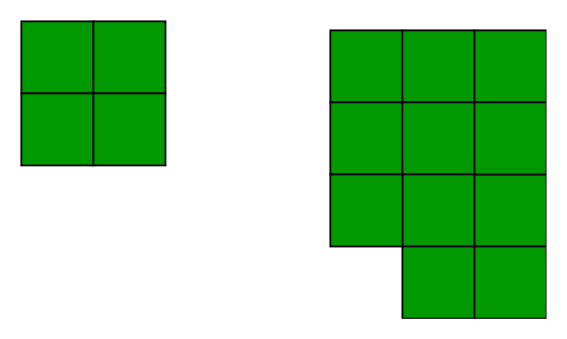Open in App
Not now

# Minimum Perimeter of n blocks

• Difficulty Level : Easy
• Last Updated : 21 Jun, 2022

We are given n blocks of size 1 x 1, we need to find the minimum perimeter of the grid made by these blocks.
Examples :

```Input : n = 4
Output : 8
Minimum possible perimeter with 4 blocks
is 8. See below explanation.

Input : n = 11
Output : 14
The square grid of above examples would be as```` `

Let us take an example to see a pattern. Let us say that we have 4 blocks, following are different possibilities

```  +--+--+--+--+
|  |  |  |  |  Perimeter = 10
+--+--+--+--+

+--+--+--+
|  |  |  |     Perimeter = 10
+--+--+--+
|  |
+--+

+--+--+--+
|  |  |  |     Perimeter = 10
+--+--+--+
|  |
+--+

+--+--+
|  |  |        Perimeter = 8
+--+--+
|  |  |
+--+--+```

If we do some examples using pen and paper, we can notice that the perimeter becomes minimum when the shape formed is closest to a square. The reason for this is, we want maximum sides of blocks to face inside the shape so that perimeter of the shape becomes minimum.
If the Number of blocks is a perfect square then the perimeter would simply be 4*sqrt(n).
But, if the Number of blocks is not a perfect square root then we calculate number of rows and columns closest to square root. After arranging the blocks in a rectangular we still have blocks left then we will simply add 2 to the perimeter because only 2 extra side would be left.
The implementation of the above idea is given below.

## C++

 `// CPP program to find minimum ` `// perimeter using n blocks.` `#include ` `using` `namespace` `std;`   `int` `minPerimeter(``int` `n)` `{` `    ``int` `l = ``sqrt``(n);` `    ``int` `sq = l * l;`   `    ``// if n is a perfect square` `    ``if` `(sq == n) ` `        ``return` `l * 4;` `    ``else` `    ``{` `        ``// Number of rows ` `        ``long` `long` `int` `row = n / l; `   `        ``// perimeter of the ` `        ``// rectangular grid ` `        ``long` `long` `int` `perimeter ` `                      ``= 2 * (l + row); `   `        ``// if there are blocks left ` `        ``if` `(n % l != 0) ` `            ``perimeter += 2;` `        ``return` `perimeter;` `    ``}` `}`   `// Driver code` `int` `main()` `{` `    ``int` `n = 10;` `    ``cout << minPerimeter(n);` `    ``return` `0;` `}`

## Java

 `// JAVA Code to find minimum ` `// perimeter using n blocks` `import` `java.util.*;`   `class` `GFG ` `{` `    ``public` `static` `long` `minPerimeter(``int` `n)` `    ``{` `        ``int` `l = (``int``) Math.sqrt(n);` `        ``int` `sq = l * l;` `    `  `        ``// if n is a perfect square` `        ``if` `(sq == n) ` `            ``return` `l * ``4``;` `        ``else` `        ``{` `            ``// Number of rows ` `            ``long` `row = n / l; ` `    `  `            ``// perimeter of the ` `            ``// rectangular grid ` `            ``long` `perimeter ` `                  ``= ``2` `* (l + row); ` `    `  `            ``// if there are blocks left ` `            ``if` `(n % l != ``0``) ` `                ``perimeter += ``2``;` `            ``return` `perimeter;` `        ``}` `    ``}` `    `  `    ``// Driver code` `    ``public` `static` `void` `main(String[] args) ` `    ``{` `        ``int` `n = ``10``;` `        ``System.out.println(minPerimeter(n));` `    ``}` `}`   `// This code is contributed by Arnav Kr. Mandal`

## Python3

 `# Python3 program to find minimum ` `# perimeter using n blocks.` `import` `math`   `def` `minPerimeter(n):` `    ``l ``=` `math.sqrt(n)` `    ``sq ``=` `l ``*` `l` ` `  `    ``# if n is a perfect square` `    ``if` `(sq ``=``=` `n): ` `        ``return` `l ``*` `4` `    ``else` `:` `        ``# Number of rows ` `        ``row ``=` `n ``/` `l` ` `  `        ``# perimeter of the ` `        ``# rectangular grid ` `        ``perimeter ``=` `2` `*` `(l ``+` `row)` `                      `  `        ``# if there are blocks left ` `        ``if` `(n ``%` `l !``=` `0``): ` `            ``perimeter ``+``=` `2` `        ``return` `perimeter`   `# Driver code` `n ``=` `10` `print``(``int``(minPerimeter(n)))`   `# This code is contributed by ` `# Prasad Kshirsagar`

## C#

 `// C# Code to find minimum ` `// perimeter using n blocks` `using` `System;`   `class` `GFG ` `{` `    ``public` `static` `long` `minPerimeter(``int` `n)` `    ``{` `        ``int` `l = (``int``) Math.Sqrt(n);` `        ``int` `sq = l * l;` `    `  `        ``// if n is a perfect square` `        ``if` `(sq == n) ` `            ``return` `l * 4;` `        ``else` `        ``{` `            ``// Number of rows ` `            ``long` `row = n / l; ` `        `  `            ``// perimeter of the ` `            ``// rectangular grid ` `            ``long` `perimeter` `                  ``= 2 * (l + row); ` `    `  `            ``// if there are blocks left ` `            ``if` `(n % l != 0) ` `                ``perimeter += 2;` `            ``return` `perimeter;` `        ``}` `    ``}` `    `  `    ``// Driver code` `    ``public` `static` `void` `Main() ` `    ``{` `        ``int` `n = 10;` `        ``Console.Write(minPerimeter(n));` `    ``}` `}`   `// This code is contributed by nitin mittal`

## PHP

 ``

## Javascript

 ``

Output :

`14`

Time complexity : O(logn)
Auxiliary Space : O(1)

References :
http://mathforum.org/library/drmath/view/61595.html
intermath.coe.uga.edu/tweb/gcsu-geo-spr06/aheath/aheath_rectperimeter.doc
This article is contributed by Sarthak Kohli. If you like GeeksforGeeks and would like to contribute, you can also write an article using write.geeksforgeeks.org or mail your article to review-team@geeksforgeeks.org. See your article appearing on the GeeksforGeeks main page and help other Geeks.